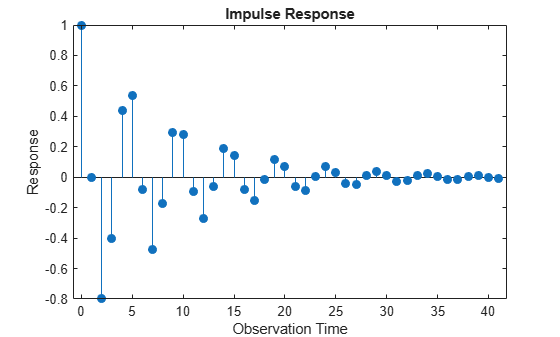Documentation

# impulse

Class: regARIMA

Impulse response of regression model with ARIMA errors

## Syntax

```impulse(Mdl) impulse(Mdl,numObs) Y = impulse(___) ```

## Description

`impulse(Mdl)` plots a discrete stem plot of the impulse response function for the regression model with ARIMA time series errors, `Mdl`, in the current figure window.

`impulse(Mdl,numObs)` plots the impulse response function for `numObs` periods.

`Y = impulse(___)` returns the impulse response in a column vector for any of the previous input arguments.

## Input Arguments

 `Mdl` Regression model with ARIMA errors, as created by `regARIMA` or `estimate`. `numObs` Number of observations to include in the impulse response, specified as a positive integer. `numObs` is the number of periods for which `impulse` computes the impulse response. Default: `impulse` determines the number of observations using the polynomial division algorithm of the underlying lag operator polynomials, `mldivide`.

## Output Arguments

 `Y` Impulse responses of the model `Mdl`, specified as a column vector. If you specify `numObs`, then `Y` is `numObs`-by-1.If you do not specify `numObs`, the underlying lag operator polynomial division algorithm returns an impulse response of generally unknown length.

## Examples

expand all

Specify the following regression model with ARMA(2,1) errors:

`$\begin{array}{l}\begin{array}{c}{y}_{t}={X}_{t}\left[\begin{array}{c}0.1\\ -0.2\end{array}\right]+{u}_{t}\\ {u}_{t}=0.5{u}_{t-1}-0.8{u}_{t-2}+{\epsilon }_{t}-0.5{\epsilon }_{t-1},\end{array}\end{array}$`

where ${\epsilon }_{t}$ is Gaussian with variance 0.1.

```Mdl = regARIMA('Intercept',0, 'AR', {0.5 -0.8}, ... 'MA',-0.5,'Beta',[0.1 -0.2], 'Variance',0.1);```

Time the calculation of and plot the impulse response function without specifying the number of observations.

```tic impulse(Mdl)````toc`
```Elapsed time is 0.356196 seconds. ```

The model is stationary; the impulse response function decays in a sinusoidal pattern.

Time the calculation of and plot the impulse response function using 45 observations.

```tic impulse(Mdl,45)````toc`
```Elapsed time is 0.164505 seconds. ```

There are more observations represented in this plot than the one generated in the previous step. However, the impulse response function and the plot took less time to generate in this step than the previous. This is because the software did not calculate the impulse response function using an infinite-degree moving average as in the previous step.

Specify the following regression model with ARMA(2,1) errors:

`$\begin{array}{l}\begin{array}{c}{y}_{t}={X}_{t}\left[\begin{array}{c}0.1\\ -0.2\end{array}\right]+{u}_{t}\\ {u}_{t}=0.5{u}_{t-1}-0.8{u}_{t-2}+{\epsilon }_{t}-0.5{\epsilon }_{t-1},\end{array}\end{array}$`

where ${\epsilon }_{t}$ is Gaussian with variance 0.1.

```Mdl = regARIMA('Intercept',0, 'AR', {0.5 -0.8}, ... 'MA',-0.5,'Beta',[0.1 -0.2], 'Variance',0.1);```

Store the impulse response function for 15 periods.

`Y = impulse(Mdl,15)`
```Y = 15×1 1.0000 0 -0.8000 -0.4000 0.4400 0.5400 -0.0820 -0.4730 -0.1709 0.2930 ⋮ ```

The length of the output impulse response series is `numObs`.

expand all

## Tips

• To improve performance of the filtering algorithm, specify the number of observations, `numObs`, to include in the impulse response.

## Algorithms

• If you specify the number of observations, `numObs`, `impulse` computes the impulse response by filtering a unit shock followed by an appropriate length vector of 0s. The filtering algorithm is very fast and results in an impulse response of known (`numObs`) length.

• If you do not specify `numObs`, then `impulse` converts the error model to a truncated, infinite-degree moving average using the relatively slow lag operator polynomial division algorithm. This produces an impulse response of generally unknown length.

## References

 Box, G. E. P., G. M. Jenkins, and G. C. Reinsel. Time Series Analysis: Forecasting and Control 3rd ed. Englewood Cliffs, NJ: Prentice Hall, 1994.

 Enders, W. Applied Econometric Time Series. Hoboken, NJ: John Wiley & Sons, 1995.

 Hamilton, J. D. Time Series Analysis. Princeton, NJ: Princeton University Press, 1994.

 Lütkepohl, H. New Introduction to Multiple Time Series Analysis. New York, NY: Springer-Verlag, 2007.